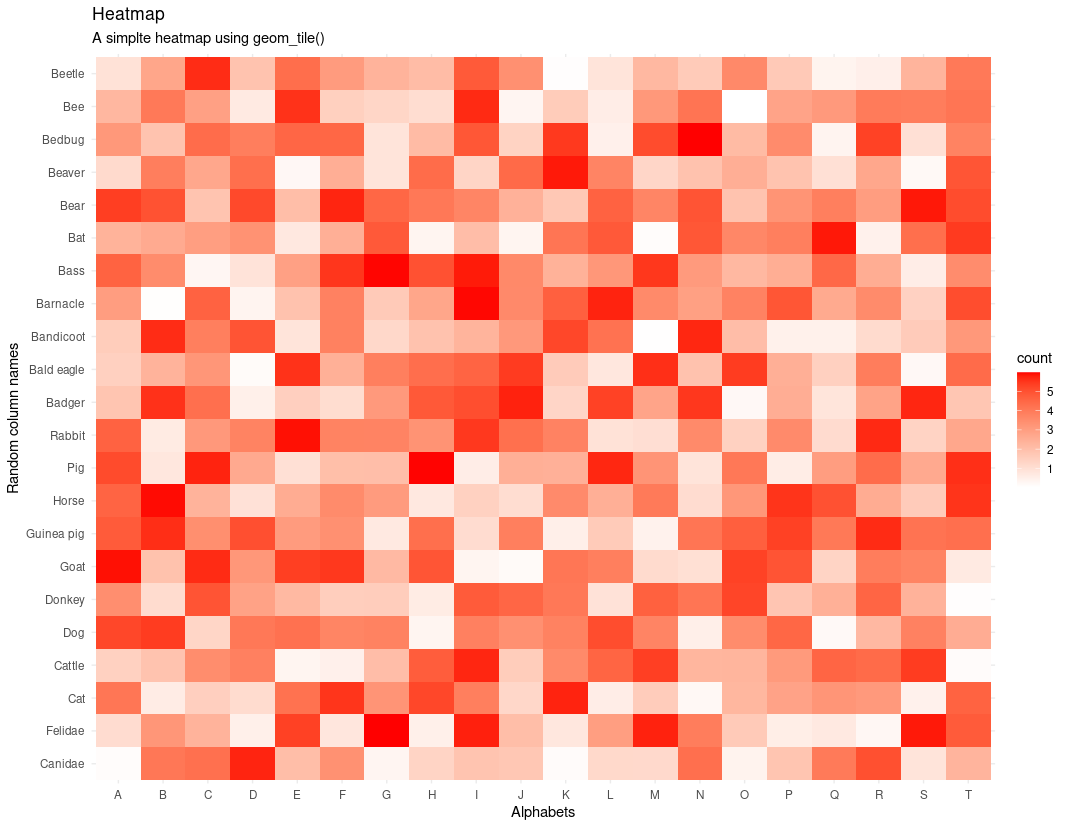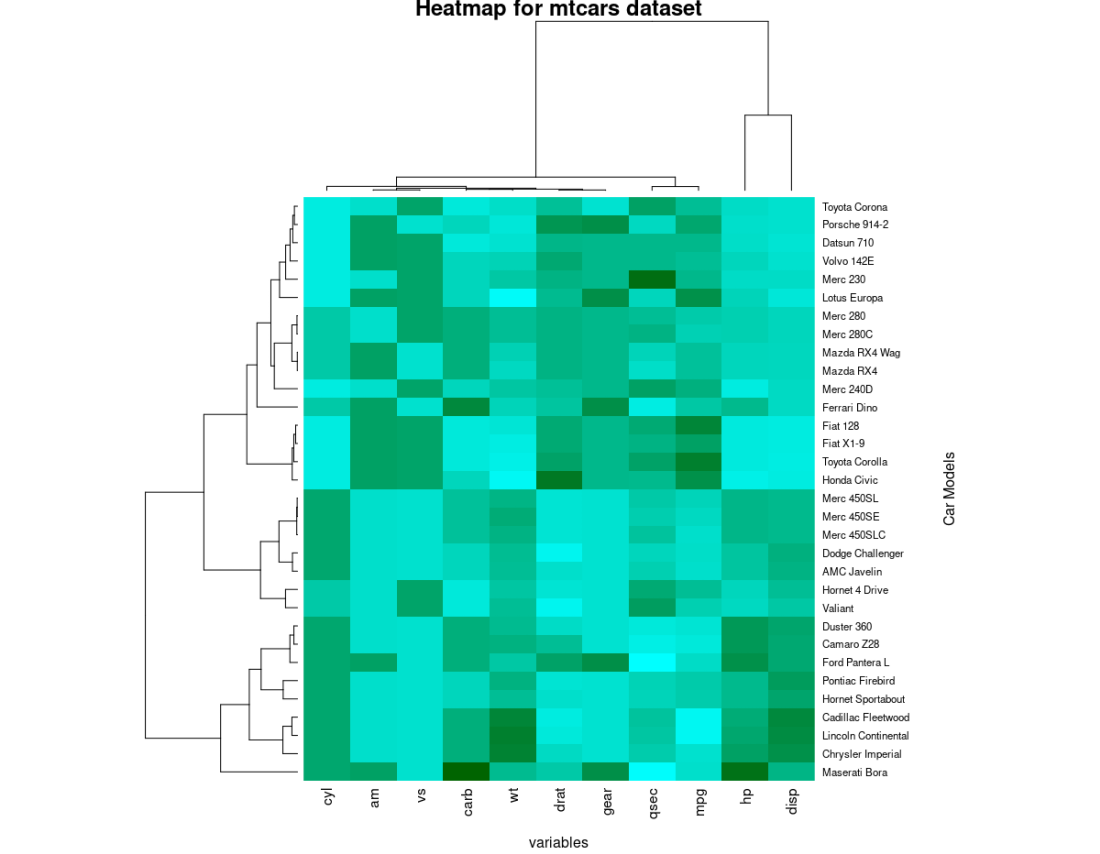# Create Heatmap in R

• Last Updated : 29 Dec, 2021

Heatmap is a graphical way to represent data. It is most commonly used in data analysis. In data analysis, we explore the dataset and draws insight from the dataset, we try to find the hidden patterns in the data by doing a visual analysis of the data. Heatmap visualizes the value of the matrix with colours, where brighter the colour means the higher the value is, and lighter the colour means the lower the value is. In Heatmap, we use warmth to cool colour scheme. Heatmap is a so-called heatmap because in heatmap we map the colours onto the different values that we have in our dataset.

In this article, we will discuss how to create heatmaps in R Programming Language.

### Method 1: Using the geom_tile() from the ggplot2 package

geom_tile() is used to create a 2-Dimensional heatmap with the plane rectangular tiles in it. It comes pre-installed with the ggplot2 package for R programming.

Syntax:

geom_tile( mapping =NULL, data=NULL, stat=”Identity”,…)

Parameter:

• mapping: It can be “aes”. “aes” is an acronym for aesthetic mapping. It describes how variables in the data are mapped to the visual properties of the geometry.
• data: It holds the dataset variable, a variable where we have stored our dataset in the script.
• stat: It is used to perform any kind of statistical operation on the dataset.

To create a heatmap, first, import the required libraries and then either create or load a dataset. Now simply call geom_tile() function with the appropriate values for parameters.

Example:

## R

 `# Plotting Heatmap in R`` ` `# adding ggplot2 library for plotting``library``(ggplot2)`` ` `# Creating random dataset``# with 20 alphabets and 20 animal names ``letters` `<- ``LETTERS``[1:20]``animal_names <- ``c``(``"Canidae"``,``"Felidae"``,``"Cat"``,``"Cattle"``,``                  ``"Dog"``,``"Donkey"``,``"Goat"``,``"Guinea pig"``,``                  ``"Horse"``,``"Pig"``,``"Rabbit"``,``"Badger"``,``                  ``"Bald eagle"``,``"Bandicoot"``,``"Barnacle"``,``                  ``"Bass"``,``"Bat"``,``"Bear"``,``"Beaver"``,``"Bedbug"``,``                  ``"Bee"``,``"Beetle"``)`` ` `data <- ``expand.grid``(X=``letters``, Y=animal_names)``data\$count <- ``runif``(440, 0, 6)`` ` `# plotting the heatmap``plt <- ``ggplot``(data,``aes``( X, Y,fill=count))``plt <- plt + ``geom_tile``()`` ` `# further customizing the heatmap by``# applying colors and title``plt <- plt + ``theme_minimal``()`` ` `# setting gradient color as red and white``plt <- plt + ``scale_fill_gradient``(low=``"white"``, high=``"red"``)`` ` `# setting the title and subtitles using``# title and subtitle``plt <- plt + ``labs``(title = ``"Heatmap"``)``plt <- plt + ``labs``(subtitle = ``"A simple heatmap using geom_tile()"``)`` ` `# setting x and y labels using labs``plt <- plt + ``labs``(x =``"Alphabets"``, y =``"Random column names"``)`` ` `# plotting the Heatmap``plt`

## R

 `# Plotting Heatmap in R`` ` `# adding ggplot2 library for plotting``library``(ggplot2)`` ` `# Creating random dataset``# with 20 alphabets and 20 animal names ``letters` `<- ``LETTERS``[1:20]``animal_names <- ``c``(``"Canidae"``,``"Felidae"``,``"Cat"``,``"Cattle"``,``                  ``"Dog"``,``"Donkey"``,``"Goat"``,``"Guinea pig"``,``                  ``"Horse"``,``"Pig"``,``"Rabbit"``,``"Badger"``,``                  ``"Bald eagle"``,``"Bandicoot"``,``"Barnacle"``,``                  ``"Bass"``,``"Bat"``,``"Bear"``,``"Beaver"``,``"Bedbug"``,``                  ``"Bee"``,``"Beetle"``)`` ` `data <- ``expand.grid``(X=``letters``, Y=animal_names)``data\$count <- ``runif``(440, 0, 6)`` ` `# plotting the heatmap``plt <- ``ggplot``(data,``aes``( X, Y,fill=count))``plt <- plt + ``geom_tile``()`` ` `# further customizing the heatmap by``# applying colors and title``plt <- plt + ``theme_minimal``()`` ` `# setting gradient color as red and white``plt <- plt + ``scale_fill_gradient``(low=``"white"``, high=``"red"``)`` ` `# setting the title and subtitles using``# title and subtitle``plt <- plt + ``labs``(title = ``"Heatmap"``)``plt <- plt + ``labs``(subtitle = ``"A simple heatmap using geom_tile()"``)`` ` `# setting x and y labels using labs``plt <- plt + ``labs``(x =``"Alphabets"``, y =``"Random column names"``)`` ` `# plotting the Heatmap``plt`

Output:Heatmap using ggplot2

### Method 2: Using heatmap() function of base R

heatmap()  function comes with the default installation of the Base R. One can use the default heatmap() also if he does not want to install any extra package. We can plot heatmap of the dataset using this heatmap function from the R.

Syntax:

heatmap(data,main = NULL, xlab = NULL, ylab = NULL,…)

Parameter:

data: data specifies the matrix of the data for which we wanted to plot a Heatmap.

main: main is a string argument, and it specifies the title of the plot.

xlab: xlab is used to specify the x-axis labels.

ylab: ylab is used to specify the y-axis labels.

Here the task is straightforward. You only need to put in the values that the function heatmap() requires.

Example:

## R

 `# Heatplot from Base R``# using default mtcars dataset from the R``x  <- ``as.matrix``(mtcars)`` ` `# custom colors``new_colors <- ``colorRampPalette``(``c``(``"cyan"``, ``"darkgreen"``)) `` ` `# plotting the heatmap``plt <- ``heatmap``(x,``               ``# assigning new colors``               ``col = ``new_colors``(100),``                ` `               ``# adding title``               ``main = ``"Heatmap for mtcars dataset"``,``                ` `               ``# adding some margin so that ``               ``# it doesn not drawn over the ``               ``# y-axis label``               ``margins = ``c``(5,10),``                ` `               ``# adding x-axis labels``               ``xlab = ``"variables"``,``                ` `               ``# adding y-axis labels``               ``ylab = ``"Car Models"``,``                ` `               ``# to scaled the values into ``               ``# column direction``               ``scale = ``"column"``)`

Output:Heatmap

My Personal Notes arrow_drop_up